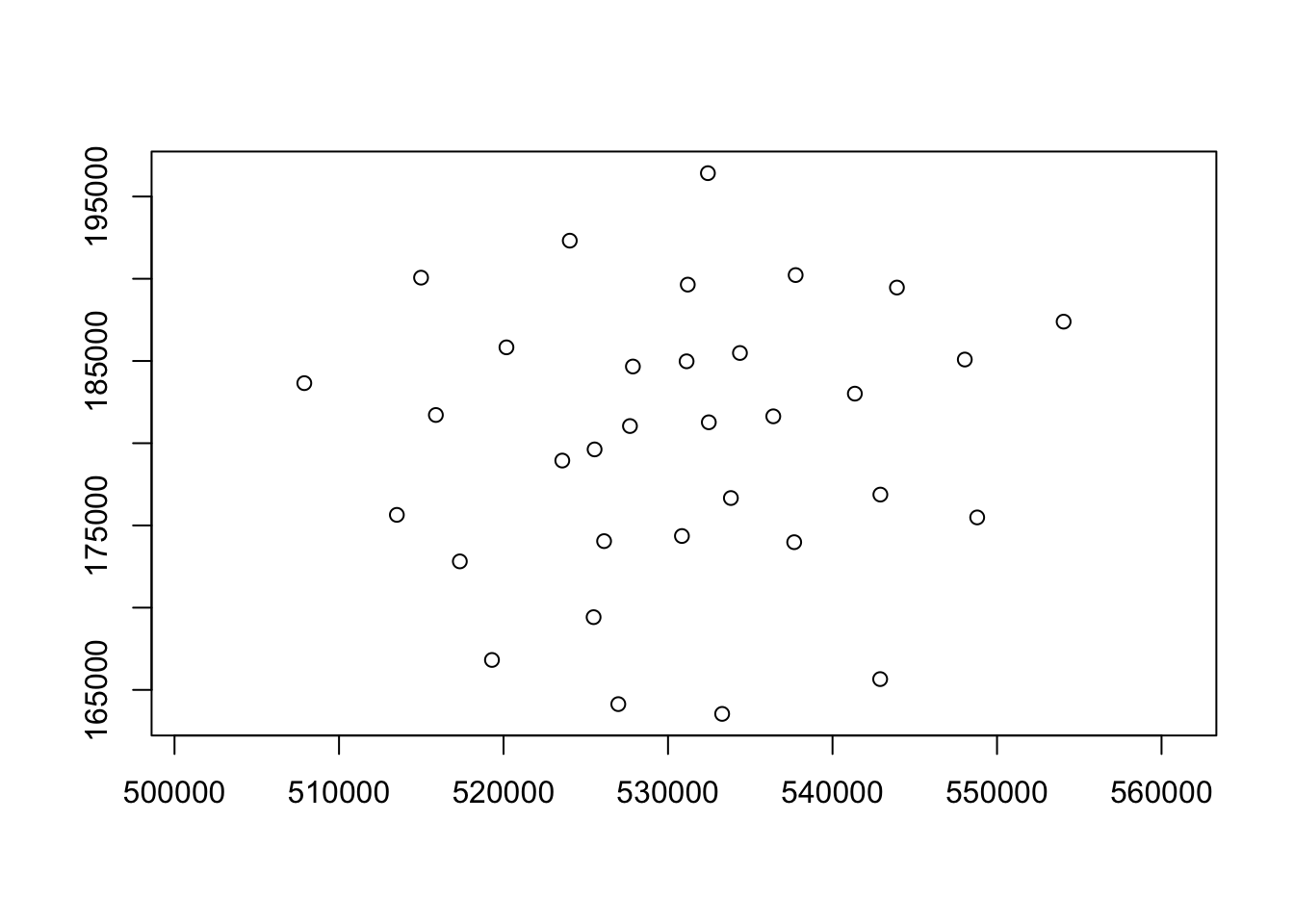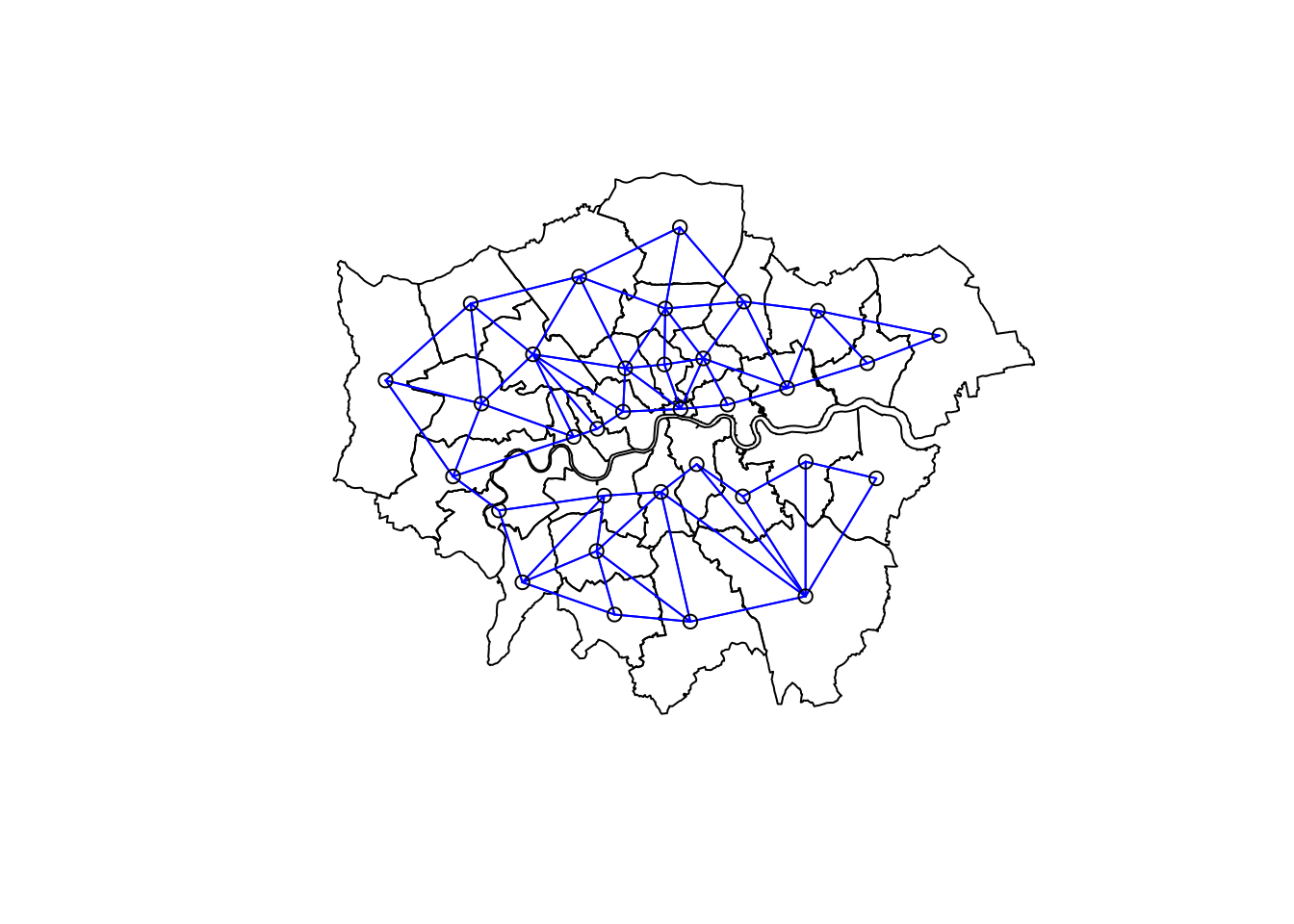# Section 7 Analysing Spatial Autocorrelation with Moran’s I

Since the sample in 2019 & 2020 is too small to run a good result, you can analysis the autocorrelation based on the samples which contain these two years.

## 7.1 Generate the data for analysis

This process is similar to the 4.2 and 4.3 data preparation.

``````sdf = merge(London_Borough,df,by="GSS_CODE",all = TRUE)
sdf = sdf[,c("GSS_CODE","geometry","longitude","latitude")]
nsdf = sdf%>%
mutate(area=st_area(.))%>%
mutate(density=n*1000*1000/area)

nsdf = dplyr::select(nsdf,density,GSS_CODE, n)

nsdf = nsdf%>%
group_by(GSS_CODE)%>%
summarise(density = first(density), GSS_CODE = first(GSS_CODE))``````
``## `summarise()` ungrouping output (override with `.groups` argument)``

## 7.2 Centroids and neighbour list

Plot the centroids of all boroughs in London

``````coordsW = nsdf%>%
st_centroid()%>%
st_geometry()``````
``````## Warning in st_centroid.sf(.): st_centroid assumes attributes are constant over
## geometries of x``````
`` plot(coordsW,axes=TRUE)``Create a neighbours list

`````` LWard_nb = nsdf %>%
poly2nb(.,queen=T)``````

Plot the neighbours list we create

``````plot(nsdf\$geometry)
plot(LWard_nb, st_geometry(coordsW), col="blue", add = T)``````Create a spatial weights object from these weights, which can contribute to the further analysis autocorrelation analysis (Moran’s I test)

``````Lward.lw = nb2listw(LWard_nb, style="C")
``````## []
##   7 12 19 30 33
##
## []
##  16 25 26
##
## []
##   5  7 10 14 15
##
## []
##   6 11
##
## []
##   3  7  9 13 15 20 33
##
## []
##   4  8 11 22 23 28``````

## 7.3 Calculate the Global Moran’I Index

Conduct the global Moran’s I test to get the value.

`````` I_LWard_Global_Density = nsdf %>%
pull(density) %>%
as.vector()%>%
moran.test(.,Lward.lw)
names(I_LWard_Global_Density)``````
``````##  "statistic"   "p.value"     "estimate"    "alternative" "method"
##  "data.name"``````
``head(I_LWard_Global_Density)``
``````## \$statistic
## Moran I statistic standard deviate
##                         0.03513093
##
## \$p.value
##  0.4859877
##
## \$estimate
## Moran I statistic       Expectation          Variance
##      -0.028283348      -0.031250000       0.007131055
##
## \$alternative
##  "greater"
##
## \$method
##  "Moran I test under randomisation"
##
## \$data.name
##  ".  \nweights: Lward.lw    \n"``````

Conduct the Local Moran’s I test in these two years

`````` I_LWard_Local_Density = nsdf %>%
pull(density) %>%
as.vector()%>%
localmoran(., Lward.lw)%>%
as_tibble()``````

Merge the moran test result with the geometric dataset. The I value is stored in “density_Iz” and the Z value is stored in “density_Iz.”

``````nsdf<-nsdf%>%
mutate(density_I = as.numeric(I_LWard_Local_Density\$Ii))%>%
mutate(density_Iz =as.numeric(I_LWard_Local_Density\$Z.Ii))
summary(nsdf\$density_I)
summary(nsdf\$density_Iz)``````

## 7.4 Interactive visulisation of the distribution of the local Moran results

For drawing an interactive map, you should set “view” variables in `tmap_mode` function. You can set break box by the minimum and maximum value of Moran.

``tmap_mode("view")``
``## tmap mode set to interactive viewing``
``````#set the group and colour
summary(nsdf\$density_Iz)``````
``````##      Min.   1st Qu.    Median      Mean   3rd Qu.      Max.
## -1.813183 -0.007425  0.133178  0.046786  0.449411  0.975067``````
``````# breaks1 = seq(-3,1,0.5)
breaks1 = c(-2,-1,-0.1,-0.01,0,0.01,0.1,0.45,1,1.5 )
# breaks2 = c(-1000,-2.58,-1.96,-1.65,1.65,1.96,2.58,1000)
# Depends on the max and min value in Moran's I
MoranColours = rev(brewer.pal(8, "RdGy"))
# Plot the map
tm_shape(nsdf) +
tm_polygons("density_Iz",
style="fixed",
breaks=breaks1,
palette=MoranColours,
midpoint=NA,
title="Local Moran's I,EV charge points in London")``````